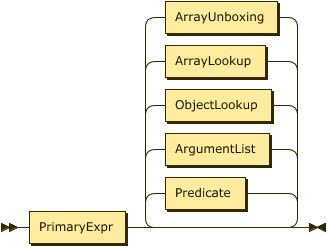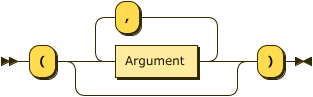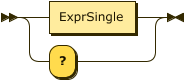### Dynamic function calls

JSONiq follows the W3C standard for dynamic function calls. The following explanations, provided as an informal summary for convenience, are non-normative.

A dynamic function call is a postfix expression. Its left-hand-side is an expression that must return a single function item (see in the data model the section called “Function items”). Its right-hand side is a list of parameters, each one of which is an arbitrary expression providing a sequence of items, one such sequence for each parameter.

Example 74. A dynamic function call.

```       let \$f := function(\$x) { \$x + 1 }
return \$f(2)
```

Result: 3

If the number of parameters does not match the arity of the function, an error is raised. An error is also raised if an argument value does not match the corresponding type in the function signature.

Otherwise, the function is evaluated with the supplied parameters. If the result matches the return type of the function, it is returned, otherwise an error is raised.

Example 75. A dynamic function call with signature

```       let \$f := function(\$x as integer) as integer { \$x + 1 }
return \$f(2)
```

Result: 3

JSONiq dynamic function calls follow the W3C specification.

Figure 40. PostfixExprFigure 41. ArgumentListFigure 42. Argument